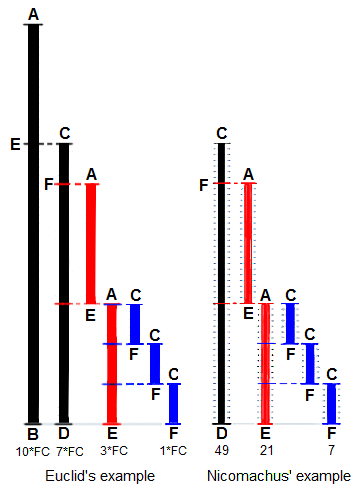## 最大公约数和Euclidean algorithm（续）

Euclidean algorithm的步骤如下图所示：1.假设$a>b$$a>b$，则令$c:=a\phantom{\rule{0.667em}{0ex}}\mathrm{mod}\phantom{\rule{thinmathspace}{0ex}}\phantom{\rule{thinmathspace}{0ex}}b$$c:=a \mod{b}$

2.如果$c=0$$c=0$，则$GCD\left(a,b\right)=b$$GCD(a,b)=b$

3.否则令$a:=b,b:=c$$a:=b,b:=c$，并返回到第1步。

Eudoxus of Cnidus，公元前390年～公元前337年，古希腊几何学家、天文学家和地理学家。柏拉图同时代最杰出的数学家。《几何原本》卷Ⅴ和卷Ⅻ主要来自欧多克索斯的工作。

Euclidean algorithm作为最古老的算法之一，被收录进Knuth的巨著TAOCP。这里的算法，指的是那些根据一定的规则来一步步执行的运算。

## Bézout’s identity和Extended Euclidean algorithm

Euclidean division还可以表示为如下形式：

$-103=-2×60+17⇒\left(-103\right)\phantom{\rule{1em}{0ex}}\mathrm{mod}\phantom{\rule{thinmathspace}{0ex}}\phantom{\rule{thinmathspace}{0ex}}60=17$

Euclidean division的定义扩展之后，则有Bézout’s identity。

Bézout’s identity：若a,b是非0整数，$d=GCD\left(a,b\right)$$d=GCD(a,b)$，则存在整数x,y使得$ax+by=d$$ax+by=d$。证明略。

${m}_{0}=3,{M}_{0}=20,\left(-1\right)20+7\left(3\right)=1$

Étienne Bézout，1730~1783，法国数学家。法国科学院院士。

$\begin{array}{}\text{(1)}& \left\{\begin{array}{l}x=1\\ y=0\end{array}\end{array}$

$a\prime x\prime +b\prime y\prime =GCD\left(a\prime ,b\prime \right)=GCD\left(b,a\phantom{\rule{1em}{0ex}}\mathrm{mod}\phantom{\rule{thinmathspace}{0ex}}\phantom{\rule{thinmathspace}{0ex}}b\right)$

$ax+by=a\prime x\prime +b\prime y\prime =bx\prime +\left(a\phantom{\rule{1em}{0ex}}\mathrm{mod}\phantom{\rule{thinmathspace}{0ex}}\phantom{\rule{thinmathspace}{0ex}}b\right)y\prime =bx\prime +\left(a-⌊\frac{a}{b}⌋b\right)y\prime$

$ax+by=ay\prime +b\left(x\prime -⌊\frac{a}{b}⌋y\prime \right)$

$\begin{array}{}\text{(2)}& \left\{\begin{array}{l}x={y}^{\prime }\\ y={x}^{\prime }-⌊\frac{a}{b}⌋y\prime \end{array}\end{array}$

https://blog.sengxian.com/algorithms/gcd-extgcd

## 中国剩余定理

Chinese remainder theorem算是初等数论中，一个非常重要的定理了。（初等数论意指使用不超过高中程度的初等代数处理的数论问题，其最主要的工具包括整数的整除性与同余。）

CRT最早出自中国四世纪成书的古书《孙子算经》。著名的娱乐圈学霸关晓彤同学所攻克的“鸡兔同笼问题”，就出自该书。

CRT的内容为：

${m}_{i}$$m_i$为两两互质（pairwise coprime）的大于1的整数，${a}_{i}$$a_i$为任意整数，则存在x满足：

$\begin{array}{}\text{(1)}& x\equiv {a}_{1}& \phantom{\rule{1em}{0ex}}\left(\mathrm{mod}\phantom{\rule{0.333em}{0ex}}{m}_{1}\right)\text{(2)}& \phantom{\rule{1em}{0ex}}⋮\text{(3)}& x\equiv {a}_{k}& \phantom{\rule{1em}{0ex}}\left(\mathrm{mod}\phantom{\rule{0.333em}{0ex}}{m}_{k}\right)\end{array}$

CRT的存在性证明略。

$\begin{array}{}\text{(4)}& x& \equiv 0\phantom{\rule{1em}{0ex}}\left(\mathrm{mod}\phantom{\rule{0.333em}{0ex}}3\right)\text{(5)}& x& \equiv 3\phantom{\rule{1em}{0ex}}\left(\mathrm{mod}\phantom{\rule{0.333em}{0ex}}4\right)\text{(6)}& x& \equiv 4\phantom{\rule{1em}{0ex}}\left(\mathrm{mod}\phantom{\rule{0.333em}{0ex}}5\right).\end{array}$

1.首先对${m}_{i}$$m_i$按降序排序。

2.选择最大的模（这里为5）和对应的${a}_{i}$$a_i$（这里为4）。

3.

{% highlight text %}
4 mod 4 → 0. Continue
4 + 5 = 9 mod 4 →1. Continue
9 + 5 = 14 mod 4 → 2. Continue
14 + 5 = 19 mod 4 → 3. OK, continue by considering remainders modulo 3 and adding 5×4 = 20 each time
19 mod 3 → 1. Continue
19 + 20 = 39 mod 3 → 0. OK, this is the result.
{% endhighlight %}

CRT虽然只是初等数论的基本定理，但应用范围很广，Lagrange interpolation（一阶多项式插值）、Hermite interpolation（多阶多项式插值）和Dedekind’s theorem，都用到了CRT。

## 多项式的Euclidean division和GCD

$\begin{array}{r}{x}^{2}+1x+3\\ x-3\overline{\right){x}^{3}-2{x}^{2}+0x-4}\\ \underset{_}{{x}^{3}-3{x}^{2}+0x-4}\\ +{x}^{2}+0x-4\\ \underset{_}{+{x}^{2}-3x-4}\\ +3x-4\\ \underset{_}{+3x-9}\\ +5\end{array}$

${x}^{3}-2{x}^{2}-4=\left(x-3\right)\phantom{\rule{thinmathspace}{0ex}}\underset{q\left(x\right)}{\underset{⏟}{\left({x}^{2}+x+3\right)}}+\underset{r\left(x\right)}{\underset{⏟}{5}}$

${x}^{2}+7x+6=\left(x+1\right)\left(x+6\right)$

${x}^{2}-5x-6=\left(x+1\right)\left(x-6\right)$

## 多项式的CRT

CRT亦可改为如下等效形式：

$c=\left(\sum _{i=0}^{k}{c}_{i}{N}_{i}{M}_{i}\right)\phantom{\rule{1em}{0ex}}\mathrm{mod}\phantom{\rule{thinmathspace}{0ex}}\phantom{\rule{thinmathspace}{0ex}}M$

${m}_{0}=3,{M}_{0}=20,\left(-1\right)20+7\left(3\right)=1$

${m}_{1}=4,{M}_{1}=15,\left(-1\right)15+\left(4\right)4=1$

${m}_{2}=5,{M}_{2}=12,\left(-2\right)12+\left(5\right)5=1$

$\begin{array}{}\text{(3)}& c\left(p\right)=\left(\sum _{i=0}^{k}{c}^{\left(i\right)}\left(p\right){N}^{\left(i\right)}\left(p\right){M}^{\left(i\right)}\left(p\right)\right)\phantom{\rule{1em}{0ex}}\mathrm{mod}\phantom{\rule{thinmathspace}{0ex}}\phantom{\rule{thinmathspace}{0ex}}M\left(p\right)\end{array}$

2x3卷积的多项式形式为：

$h\left(p\right)={h}_{0}+{h}_{1}p,x\left(p\right)={x}_{0}+{x}_{1}p+{x}_{2}{p}^{2},s\left(p\right)=h\left(p\right)x\left(p\right)$

Step 1：首先要构造一个degree大于等于3的多项式：

$m\left(p\right)={m}^{\left(0\right)}\left(p\right){m}^{\left(0\right)}\left(p\right)\cdots {m}^{\left(k\right)}\left(p\right)$

i
${m}^{\left(i\right)}\left(p\right)$
${M}^{\left(i\right)}\left(p\right)$
${n}^{\left(i\right)}\left(p\right)$
${N}^{\left(i\right)}\left(p\right)$
0
$p$
${p}^{2}-1$
$p$
$-1$
1
$p-1$
${p}^{2}+p$
$-\frac{1}{2}\left(p+2\right)$
$\frac{1}{2}$
2
$p+1$
${p}^{2}-p$
$-\frac{1}{2}\left(p-2\right)$
$\frac{1}{2}$

Step 2：使用如下公式计算${h}^{\left(i\right)}\left(p\right),{x}^{\left(i\right)}\left(p\right)$$h^{(i)}(p),x^{(i)}(p)$

${h}^{\left(i\right)}\left(p\right)=h\left(p\right)\phantom{\rule{1em}{0ex}}\mathrm{mod}\phantom{\rule{thinmathspace}{0ex}}\phantom{\rule{thinmathspace}{0ex}}{m}^{\left(i\right)}\left(p\right)$

${x}^{\left(i\right)}\left(p\right)=x\left(p\right)\phantom{\rule{1em}{0ex}}\mathrm{mod}\phantom{\rule{thinmathspace}{0ex}}\phantom{\rule{thinmathspace}{0ex}}{m}^{\left(i\right)}\left(p\right)$

${h}^{\left(0\right)}\left(p\right)={h}_{0},{x}^{\left(0\right)}\left(p\right)={x}_{0}$

${h}^{\left(1\right)}\left(p\right)={h}_{0}+{h}_{1},{x}^{\left(1\right)}\left(p\right)={x}_{0}+{x}_{1}+{x}_{2}$

${h}^{\left(2\right)}\left(p\right)={h}_{0}-{h}_{1},{x}^{\left(2\right)}\left(p\right)={x}_{0}-{x}_{1}+{x}_{2}$

Step 3：使用如下公式计算${s}^{\prime \left(i\right)}\left(p\right)$$s'^{(i)}(p)$

${s}^{\prime \left(i\right)}\left(p\right)={h}^{\left(i\right)}\left(p\right){x}^{\left(i\right)}\left(p\right)\phantom{\rule{1em}{0ex}}\mathrm{mod}\phantom{\rule{thinmathspace}{0ex}}\phantom{\rule{thinmathspace}{0ex}}{m}^{\left(i\right)}\left(p\right)$

${s}^{\prime \left(0\right)}\left(p\right)={h}_{0}{x}_{0}$

${s}^{\prime \left(1\right)}\left(p\right)=\left({h}_{0}+{h}_{1}\right)\left({x}_{0}+{x}_{1}+{x}_{2}\right)$

${s}^{\prime \left(2\right)}\left(p\right)=\left({h}_{0}-{h}_{1}\right)\left({x}_{0}-{x}_{1}+{x}_{2}\right)$

Step 4：根据公式3计算余数${s}^{\prime }\left(p\right)$$s'(p)$，并利用如下公式计算被除数$s\left(p\right)$$s(p)$

$s\left(p\right)={s}^{\prime }\left(p\right)+{h}_{N-1}{x}_{L-1}m\left(p\right)$

$\begin{array}{}\text{(13)}& s\left(p\right)& ={s}^{\prime }\left(p\right)+{h}_{1}{x}_{2}m\left(p\right)\text{(14)}& & ={s}^{\prime }\left(0\right)\left(-{p}^{2}+1\right)+\frac{{s}^{\prime }\left(1\right)}{2}\left({p}^{2}+p\right)+\frac{{s}^{\prime }\left(2\right)}{2}\left({p}^{2}-p\right)+{h}_{1}{x}_{2}m\left({p}^{3}-p\right)\text{(15)}& & ={s}^{\prime }\left(0\right)+p\left(\frac{{s}^{\prime }\left(1\right)}{2}-\frac{{s}^{\prime }\left(2\right)}{2}-{h}_{1}{x}_{2}\right)+{p}^{2}\left(-{s}^{\prime }\left(0\right)+\frac{{s}^{\prime }\left(1\right)}{2}+\frac{{s}^{\prime }\left(2\right)}{2}\right)+{p}^{3}\left({h}_{1}{x}_{2}\right)\end{array}$

《Fast Algorithms for Convolutional Neural Networks》

https://github.com/andravin/wincnn

## FFT与卷积

FFT是加速卷积运算的一种常用方法。但由于其原理，当卷积核小的时候，是没什么加速的，当核是3或者5时，速度有时更慢或者相当，而在CNN中卷积的核大多数比较小，FFT起到的加速作用很小，所以基本没人用。

http://www.cnblogs.com/jianyingzhou/p/4303578.html

convolution,fft, 加速

## 参考

https://colfaxresearch.com/falcon-library/

FALCON Library: Fast Image Convolution in Neural Networks on Intel Architecture

10-121万+

12-112万+05-121842

06-111万+

#### 深度学习（二十六）——VAE

07-07134

#### 深度学习算法优化系列 | Google CVPR2018 int8量化算法

07-23254

#### NLP：MRC常用数据集

05-287637

#### 深度学习（二十四）——L2 Normalization, Attention（1）

12-051万+

#### 机器学习（十二）——机器学习中的矩阵方法（2）特征值和奇异值

06-013297

04-099389

#### 深度学习（二十一）——SRCNN, DRCN, VDSR©️2020 CSDN 皮肤主题: 大白 设计师: CSDN官方博客点击重新获取扫码支付1.余额是钱包充值的虚拟货币，按照1:1的比例进行支付金额的抵扣。
2.余额无法直接购买下载，可以购买VIP、C币套餐、付费专栏及课程。余额充值